Anzeige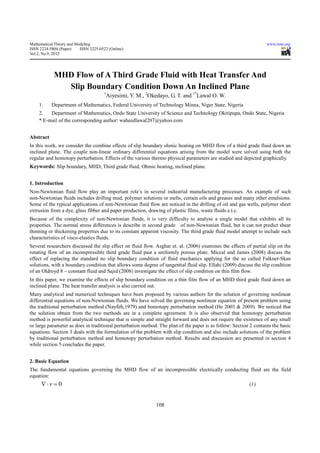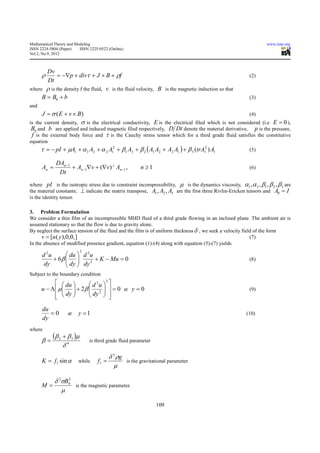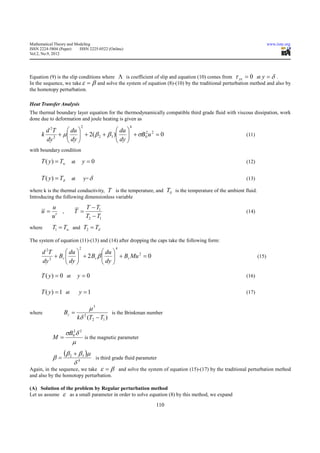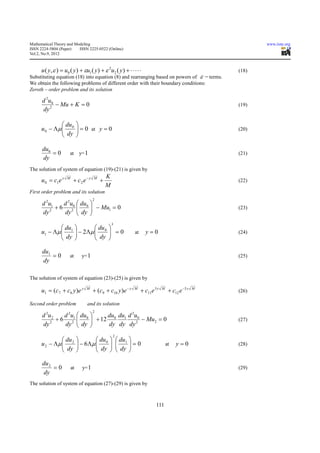Anzeige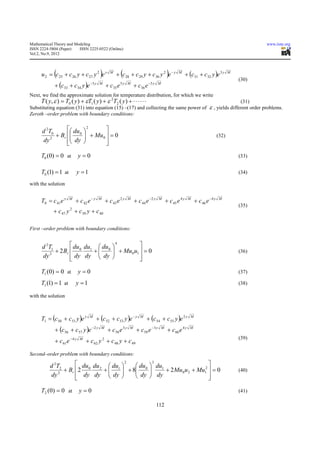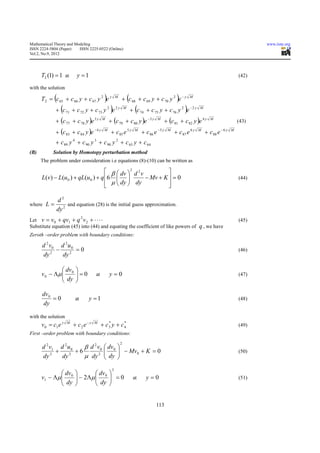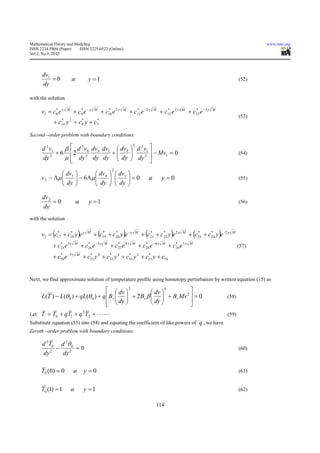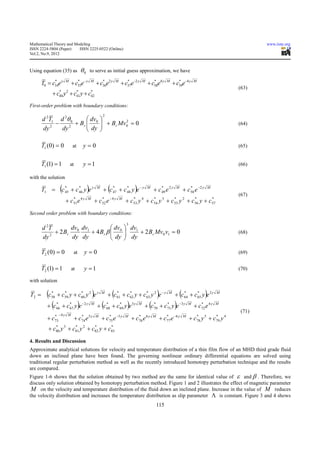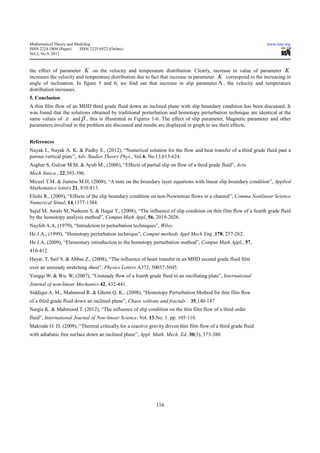Anzeige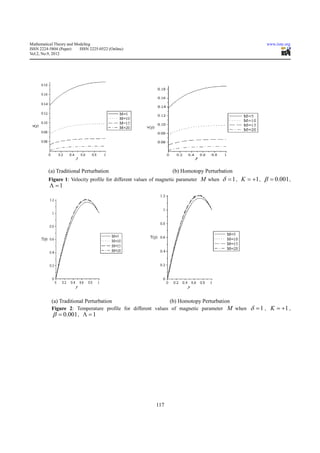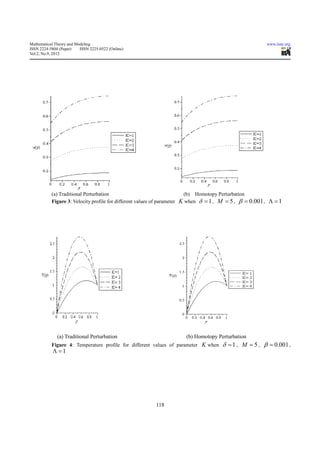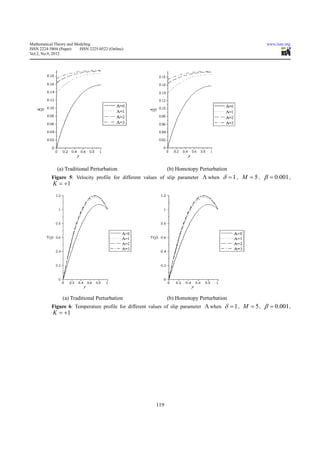Nächste SlideShare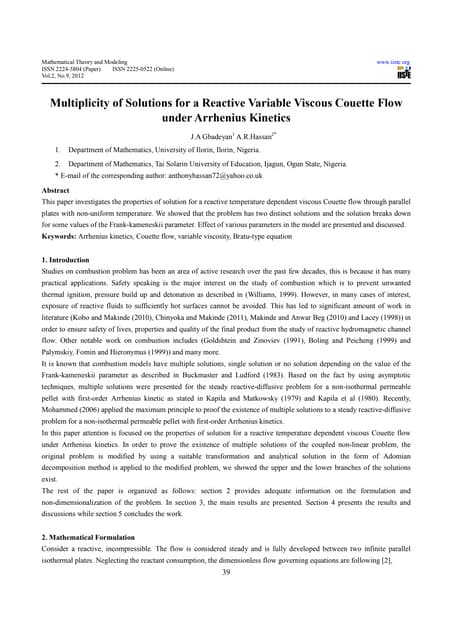Multiplicity of solutions for a reactive variable viscous couette flow
1 von 13
Anzeige

### Mhd flow of a third grade fluid with heat transfer and

1. Mathematical Theory and Modeling www.iiste.org ISSN 2224-5804 (Paper) ISSN 2225-0522 (Online) Vol.2, No.9, 2012 MHD Flow of A Third Grade Fluid with Heat Transfer And Slip Boundary Condition Down An Inclined Plane 1 Aiyesimi, Y. M., 2Okedayo, G. T. and 1*Lawal O. W. 1. Department of Mathematics, Federal University of Technology Minna, Niger State, Nigeria 2. Department of Mathematics, Ondo State University of Science and Technology Okitipupa, Ondo State, Nigeria * E-mail of the corresponding author: waheedlawal207@yahoo.com Abstract In this work, we consider the combine effects of slip boundary ohmic heating on MHD flow of a third grade fluid down an inclined plane. The couple non-linear ordinary differential equations arising from the model were solved using both the regular and homotopy perturbation. Effects of the various thermo physical parameters are studied and depicted graphically. Keywords: Slip boundary, MHD, Third grade fluid, Ohmic heating, inclined plane. 1. Introduction Non-Newtonian fluid flow play an important role’s in several industrial manufacturing processes. An example of such non-Newtonian fluids includes drilling mud, polymer solutions or melts, certain oils and greases and many other emulsions. Some of the typical applications of non-Newtonian fluid flow are noticed in the drilling of oil and gas wells, polymer sheet extrusion from a dye, glass fibber and paper production, drawing of plastic films, waste fluids e.t.c. Because of the complexity of non-Newtonian fluids, it is very difficulty to analyse a single model that exhibits all its properties. The normal stress differences is describe in second grade of non-Newtonian fluid, but it can not predict shear thinning or thickening properties due to its constant apparent viscosity. The third grade fluid model attempt to include such characteristics of visco-elastics fluids. Several researchers discussed the slip effect on fluid flow. Asghar et. al. (2006) examines the effects of partial slip on the rotating flow of an incompressible third grade fluid past a uniformly porous plate. Miccal and James (2008) discuss the effect of replacing the standard no slip boundary condition of fluid mechanics applying for the so called Falkner-Skan solutions, with a boundary condition that allows some degree of tangential fluid slip. Ellahi (2009) discuss the slip condition of an Oldroyd 8 – constant fluid and Sajid (2008) investigate the effect of slip condition on thin film flow. In this paper, we examine the effects of slip boundary condition on a thin film flow of an MHD third grade fluid down an inclined plane. The heat transfer analysis is also carried out. Many analytical and numerical techniques have been proposed by various authors for the solution of governing nonlinear differential equations of non-Newtonian fluids. We have solved the governing nonlinear equation of present problem using the traditional perturbation method (Nayfeh,1979) and homotopy perturbation method (He 2003 & 2009). We noticed that the solution obtain from the two methods are in a complete agreement. It is also observed that homotopy perturbation method is powerful analytical technique that is simple and straight forward and does not require the existence of any small or large parameter as does in traditional perturbation method. The plan of the paper is as follow: Section 2 contains the basic equations. Section 3 deals with the formulation of the problem with slip condition and also include solutions of the problem by traditional perturbation method and homotopy perturbation method. Results and discussion are presented in section 4 while section 5 concludes the paper. 2. Basic Equation The fundamental equations governing the MHD flow of an incompressible electrically conducting fluid are the field equation: ∇⋅v = 0 (1) 108
2. Mathematical Theory and Modeling www.iiste.org ISSN 2224-5804 (Paper) ISSN 2225-0522 (Online) Vol.2, No.9, 2012 Dv ρ = −∇p + divτ + J × B + ρf (2) Dt where ρ is the density f the fluid, v is the fluid velocity, B is the magnetic induction so that B = B0 + b (3) and J = σ ( E + v × B) (4) is the current density, σ is the electrical conductivity, E is the electrical filed which is not considered (i.e E = 0 ), B0 and b are applied and induced magnetic filed respectively, D Dt denote the material derivative, p is the pressure, f is the external body force and τ is the Cauchy stress tensor which for a third grade fluid satisfies the constitutive equation τ = − pI + µA1 + α 1 A2 + α 2 A12 + β1 A3 + β 2 ( A1 A2 + A2 A1 ) + β 3 (trA12 ) A1 (5) DAn −1 An = + An−1∇v + (∇v) ⊥ An −1 , n ≥1 (6) Dt where pI is the isotropic stress due to constraint incompressibility, µ is the dynamics viscosity, α1 , α 2 , β1 , β 2 , β 3 are the material constants; ⊥ indicate the matrix transpose, A1 , A2 , A3 are the first three Rivlin-Ericken tensors and A0 = I is the identity tensor. 3. Problem Formulation We consider a thin film of an incompressible MHD fluid of a third grade flowing in an inclined plane. The ambient air is assumed stationary so that the flow is due to gravity alone. By neglect the surface tension of the fluid and the film is of uniform thickness δ , we seek a velocity field of the form v = [u ( y ),0,0, ] (7) In the absence of modified presence gradient, equation (1)-(4) along with equation (5)-(7) yields 2 d 2u  du  d 2 u + 6β    dy  dy 2 + K − Mu = 0 (8) dy   Subject to the boundary condition   du   d 2u   3 u − Λ  µ   + 2 β  2   = 0 at y = 0    dy  (9)   dy      du =0 at y =1 (10) dy where β= (β 2 + β 3 )µ is third grade fluid parameter δ4 δ 3 ρg K = f1 sin α while f1 = is the gravitational parameter. µ δ 2σB02 M= is the magnetic parameter. µ 109
3. Mathematical Theory and Modeling www.iiste.org ISSN 2224-5804 (Paper) ISSN 2225-0522 (Online) Vol.2, No.9, 2012 Equation (9) is the slip conditions where Λ is coefficient of slip and equation (10) comes from τ yx = 0 at y = δ . In the sequence, we take ε = β and solve the system of equation (8)-(10) by the traditional perturbation method and also by the homotopy perturbation. Heat Transfer Analysis The thermal boundary layer equation for the thermodynamically compatible third grade fluid with viscous dissipation, work done due to deformation and joule heating is given as 2 4 d 2T  du   du  k 2 + µ   + 2( β 2 + β 3 )  + σB02 u 2 = 0    dy  (11) dy  dy    with boundary condition T ( y ) = Tw at y=0 (12) T ( y ) = Tδ at y= δ (13) where k is the thermal conductivity, T is the temperature, and Tδ is the temperature of the ambient fluid. Introducing the following dimensionless variable u T − T1 u= , T = (14) u′ T2 − T1 where T1 = Tw and T2 = Tδ The system of equation (11)-(13) and (14) after dropping the caps take the following form: 2 4 d 2T  du   du  2 + Br   + 2 Br β   + Br Mu 2 = 0    dy  (15) dy  dy    T ( y ) = 0 at y=0 (16) T ( y ) = 1 at y =1 (17) µ3 where Br = is the Brinkman number kδ 2 (T2 − T1 ) σB02δ 2 M= is the magnetic parameter µ β= (β 2 + β 3 )µ is third grade fluid parameter δ4 Again, in the sequence, we take ε = β and solve the system of equation (15)-(17) by the traditional perturbation method and also by the homotopy perturbation. (A) Solution of the problem by Regular perturbation method Let us assume ε as a small parameter in order to solve equation (8) by this method, we expand 110
4. Mathematical Theory and Modeling www.iiste.org ISSN 2224-5804 (Paper) ISSN 2225-0522 (Online) Vol.2, No.9, 2012 u ( y, ε ) = u0 ( y ) + εu1 ( y ) + ε 2u2 ( y ) + ⋅ ⋅ ⋅ ⋅ ⋅ (18) Substituting equation (18) into equation (8) and rearranging based on powers of ε − terms. We obtain the following problems of different order with their boundary conditions: Zeroth – order problem and its solution d 2u 0 − Mu + K = 0 (19) dy 2  du  u 0 − Λµ  0  dy  = 0 at y = 0  (20)   du0 =0 at y= 1 (21) dy The solution of system of equation (19)-(21) is given by K u 0 = c1e y M + c2 e − y M + (22) M First order problem and its solution 2 d 2u1 d 2u  du  + 6 20  0  − Mu1 = 0 (23) dy 2 dy  dy    3  du   du  u1 − Λµ  1  − 2Λµ  0  dy   dy  =0  at y=0 (24)     du1 =0 at y= 1 (25) dy The solution of system of equation (23)-(25) is given by u1 = (c7 + c8 y )e y M + (c9 + c10 y )e − y M + c11e 3 y M + c12 e −3 y M (26) Second order problem and its solution 2 d 2u 2 d 2u  du  du du d 2u0 + 6 21  0  + 12 0 1 − Mu2 = 0 (27) dy 2 dy  dy    dy dy dy 2 2  du   du   du1  u 2 − Λµ  2  dy  − 6 Λµ  0   dy     dy  = 0  at y=0 (28)       du 2 =0 at y= 1 (29) dy The solution of system of equation (27)-(29) is given by 111
5. Mathematical Theory and Modeling www.iiste.org ISSN 2224-5804 (Paper) ISSN 2225-0522 (Online) Vol.2, No.9, 2012 ( u 2 = c 25 + c 26 y + c 27 y 2 e y ) M ( + c28 + c 29 y + c30 y 2 e − y ) M + (c31 + c32 y )e 3 y M (30) + (c33 + c34 y )e −3 y M + c35 e 5 y M + c36 e −5 y M Next, we find the approximate solution for temperature distribution, for which we write T ( y, ε ) = T0 ( y ) + εT1 ( y ) + ε 2T2 ( y ) + ⋅ ⋅ ⋅ ⋅ ⋅ ⋅ (31) Substituting equation (31) into equation (15) –(17) and collecting the same power of ε , yields different order problems. Zeroth –order problem with boundary conditions: d 2T0  du  2  + Br  0  + Mu 0  = 0   (32) dy 2  dy     T0 (0) = 0 at y=0 (33) T0 (1) = 1 at y =1 (34) with the solution T0 = c 41 e y M + c 42 e − y M + c 43 e 2 y M + c 44 e −2 y M + c 45 e 4 y M + c 46 e −4 y M (35) + c 47 y 2 + c39 y + c 40 First –order problem with boundary conditions: d 2T1  du du  du  4  + 2 Br  0 1 +  0  + Mu 0 u1  = 0   (36) dy 2  dy dy  dy     T1 (0) = 0 at y=0 (37) T1 (1) = 1 at y =1 (38) with the solution T1 = (c50 + c51 y )e y M + (c 52 + c 53 y )e − y M + (c 54 + c 55 y )e 2 y M + (c56 + c57 y )e − 2 y M + c58 e 3 y M + c59 e −3 y M + c 60 e 4 y M (39) + c 61 e − 4 y M + c 62 y 2 + c 48 y + c 49 Second–order problem with boundary conditions: d 2T2  du du  du  2  du  3 du  2 + Br 2 0 2 +  1  + 8 0   dy   dy  dy + 2 Mu 0 u 2 + Mu1  = 0 1 2 (40) dy  dy dy        T2 (0) = 0 at y=0 (41) 112
6. Mathematical Theory and Modeling www.iiste.org ISSN 2224-5804 (Paper) ISSN 2225-0522 (Online) Vol.2, No.9, 2012 T2 (1) = 1 at y =1 (42) with the solution ( T 2 = c 65 + c 66 y + c 67 y 2 e y ) M ( + c 68 + c 69 y + c 70 y 2 e − y ) M ( + c 71 + c 72 y + c 73 y 2 e 2 y ) M ( + c 74 + c 75 y + c 76 y 2 e − 2 y) M + (c 77 + c 78 y )e 3 y M + (c 79 + c 80 y )e − 3 y M + (c 81 + c 82 y )e 4 y M (43) + (c 83 + c 84 y )e − 4 y M + c 85 e 5 y M + c 86 e − 5 y M + c 87 e 6 y M + c 88 e − 6 y M + c 89 y 4 + c 90 y 3 + c 90 y 2 + c 63 y + c 64 (B) Solution by Homotopy perturbation method The problem under consideration i.e equations (8)-(10) can be written as  β  dv  2 d 2 v  L(v) − L(u 0 ) + qL(u 0 ) + q 6     − Mv + K  = 0 (44)  µ  dy  dy    d2 where L= and equation (28) is the initial guess approximation. dy 2 Let v = v 0 + qv1 + q v 2 + ⋅ ⋅ ⋅ ⋅ 2 (45) Substitute equation (45) into (44) and equating the coefficient of like powers of q , we have Zeroth –order problem with boundary conditions: d 2 v0 d 2 u 0 − =0 (46) dy 2 dy 2  dv  v 0 − Λµ  0  dy =0  at y=0 (47)   dv 0 =0 at y =1 (48) dy with the solution v 0 = c1e y M + c2 e − y M + c3 y + c 4 * * (49) First –order problem with boundary conditions: 2 d 2 v1 d 2 u 0 β d 2 v0  dv0  + +6   dy  − Mv0 + K = 0  (50) dy 2 dy 2 µ dy 2   3  dv   dv  v1 − Λµ  0  dy  − 2 Λµ  0   dy  =0  at y=0 (51)     113
7. Mathematical Theory and Modeling www.iiste.org ISSN 2224-5804 (Paper) ISSN 2225-0522 (Online) Vol.2, No.9, 2012 dv1 =0 at y =1 (52) dy with the solution v1 = c8 e y * M + c9 e − y * M + c10 e 2 y * M + c11 e −2 y * M + c12 e 3 y * M + c13 e −3 y * M (53) + c14 y 2 + c 6 y + c 7 * * * Second –order problem with boundary conditions: β  d 2 v dv dv  dv  d 2 v1  2 d 2 v1 + 6 2 20 0 1 +  0   dy 2  − Mv1 = 0 (54) dy 2 µ  dy dy dy  dy      2  dv   dv   dv1  v 2 − Λµ  1  − 6Λµ  0  dy   dy     dy  = 0  at y=0 (55)       dv 2 =0 at y =1 (56) dy with the solution ( v2 = c17 + c18 y e y * * ) M * ( + c19 + c20 y e − y * ) M ( + c21 + c22 y e 2 y * * ) M * ( * ) + c23 + c24 y e −2 y M + c25 e 3 y * M + c26 e −3 y * M + c27 e 4 y * M + c28 e −4 y * M + c29 e 5 y * M (57) + c30 e −5 y * M + c31 y 4 + c32 y 3 + c33 y 2 + c15 y + c16 * * * * Next, we find approximate solution of temperature profile using homotopy perturbation by written equation (15) as   dv  2  dv  4  L(T ) − L(θ 0 ) + qL(θ 0 ) + q  Br   + 2 Br β   + Br Mv 2  = 0  dy    (58)      dy    Let T = T0 + qT1 + q T2 + ⋅ ⋅ ⋅ ⋅ ⋅ ⋅ 2 (59) Substitute equation (55) into (54) and equating the coefficient of like powers of q , we have Zeroth –order problem with boundary conditions: d 2T0 d 2θ 0 − =0 (60) dy 2 dy 2 T0 (0) = 0 at y=0 (61) T0 (1) = 1 at y =1 (62) 114
8. Mathematical Theory and Modeling www.iiste.org ISSN 2224-5804 (Paper) ISSN 2225-0522 (Online) Vol.2, No.9, 2012 Using equation (35) as θ0 to serve as initial guess approximation, we have T0 = c34e y * M + c35e− y * M + c36e2 y * M + c37e−2 y * M + c38e4 y * M + c39e−4 y * M (63) + c40 y2 + c41 y + c42 * * * First-order problem with boundary conditions: 2 d 2T1 d 2θ 0  dv  2 − 2 + Br  0  dy  + Br Mv0 = 0  2 (64) dy dy   T1 (0) = 0 at y=0 (65) T1 (1) = 1 at y =1 (66) with the solution T1 = (c * 45 + c 46 y e y * ) M ( + c 47 + c 48 y e − y * * ) M + c 49 e 2 y * M + c50 e −2 y * M (67) + c 51 e 4 y * M + c 52 e − 4 y * M + c53 y 4 + c 54 y 3 + c 55 y 2 + c56 y + c57 * * * * * Second order problem with boundary conditions: 3 d 2T dv dv  dv  dv1 2 + 2 Br 0 1 + 4 B r β  0  dy   dy + 2 Br Mv0 v1 = 0 (68) dy dy dy   T2 (0) = 0 at y=0 (69) T2 (1) = 1 at y =1 (70) with solution T2 = (c * 58 + c59 y + c60 y 2 e y * * ) M * ( + c61 + c62 y + c63 y 2 e − y * * ) M * ( + c64 + c65 y e 2 y * ) M ( + c66 + c67 y e −2 y * * ) M * ( + c68 + c69 y e 3 y * ) M * ( + c70 + c71 y e −3 y * ) M + c72 e 4 y * M (71) −4 y M + c73 * + c74 e 5 y * M + c75 e −5 y * M + c76 e 6 y * M + c77 e −6 y * M + c78 y 5 + c79 y 4 * * + c80 y 3 + c81 y 2 + c82 y + c83 * * * * 4. Results and Discussion Approximate analytical solutions for velocity and temperature distribution of a thin film flow of an MHD third grade fluid down an inclined plane have been found. The governing nonlinear ordinary differential equations are solved using traditional regular perturbation method as well as the recently introduced homotopy perturbation technique and the results are compared. Figure 1-6 shows that the solution obtained by two method are the same for identical value of ε and β . Therefore, we discuss only solution obtained by homotopy perturbation method. Figure 1 and 2 illustrates the effect of magnetic parameter M on the velocity and temperature distribution of the fluid down an inclined plane. Increase in the value of M reduces the velocity distribution and increases the temperature distribution as slip parameter Λ is constant. Figure 3 and 4 shows 115